# Introduction to Evolutionary Computation Evolutionary Computation is the

• Slides: 21
Download presentationIntroduction to Evolutionary Computation • Evolutionary Computation is the field of study devoted to the design, development, and analysis is problem solvers based on natural selection (simulated evolution). • Evolution has proven to be a powerful search process. • Evolutionary Computation has been successfully applied to a wide range of problems including: – – Aircraft Design, Routing in Communications Networks, Tracking Windshear, Game Playing (Checkers [Fogel])Introduction to Evolutionary Computation (Applications cont. ) • • • Robotics, Air Traffic Control, Design, Scheduling, Machine Learning, Pattern Recognition, Job Shop Scheduling, VLSI Circuit Layout, Strike Force Allocation,Introduction to Evolutionary Computation (Applications cont. ) • Theme Park Tours (Disney Land/World) http: //www. Touring. Plans. com • Market Forecasting, • Egg Price Forecasting, • Design of Filters and Barriers, • Data-Mining, • User-Mining, • Resource Allocation, • Path Planning, • Etc.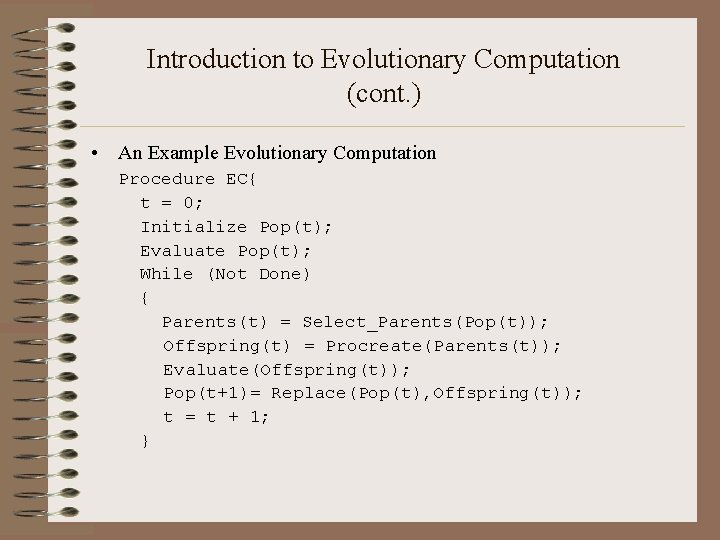Introduction to Evolutionary Computation (cont. ) • An Example Evolutionary Computation Procedure EC{ t = 0; Initialize Pop(t); Evaluate Pop(t); While (Not Done) { Parents(t) = Select_Parents(Pop(t)); Offspring(t) = Procreate(Parents(t)); Evaluate(Offspring(t)); Pop(t+1)= Replace(Pop(t), Offspring(t)); t = t + 1; }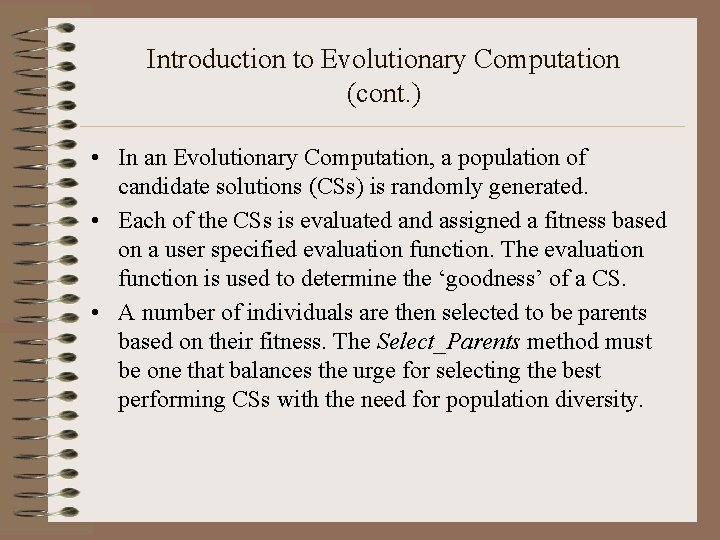Introduction to Evolutionary Computation (cont. ) • In an Evolutionary Computation, a population of candidate solutions (CSs) is randomly generated. • Each of the CSs is evaluated and assigned a fitness based on a user specified evaluation function. The evaluation function is used to determine the ‘goodness’ of a CS. • A number of individuals are then selected to be parents based on their fitness. The Select_Parents method must be one that balances the urge for selecting the best performing CSs with the need for population diversity.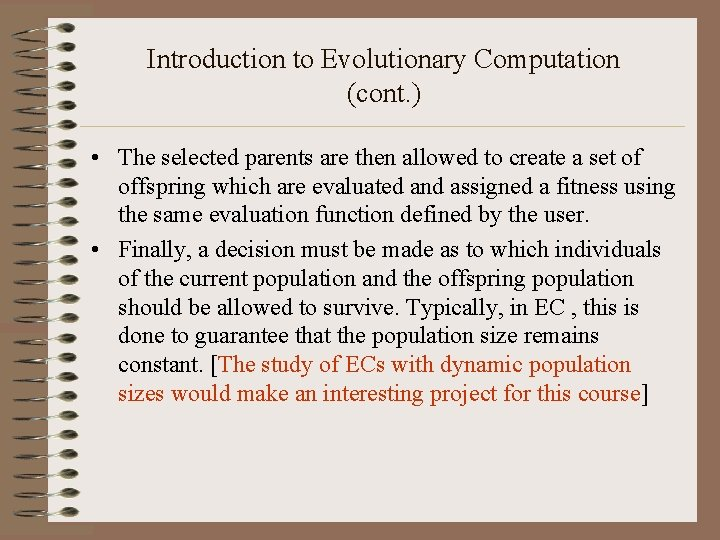Introduction to Evolutionary Computation (cont. ) • The selected parents are then allowed to create a set of offspring which are evaluated and assigned a fitness using the same evaluation function defined by the user. • Finally, a decision must be made as to which individuals of the current population and the offspring population should be allowed to survive. Typically, in EC , this is done to guarantee that the population size remains constant. [The study of ECs with dynamic population sizes would make an interesting project for this course]Introduction to Evolutionary Computation (cont. ) • Once a decision is made the survivors comprise the next generation (Pop(t+1)). • This process of selecting parents based on their fitness, allowing them to create offspring, and replacing weaker members of the population is repeated for a user specified number of cycles. • Stopping conditions for evolutionary search could be: – – – The discovery of an optimal or near optimal solution Convergence on a single solution or set of similar solutions, When the EC detects the problem has no feasible solution, After a user-specified threshold has been reached, or After a maximum number of cycles.A Brief History of Evolutionary Computation • The idea of using simulated evolution to solve engineering and design problems have been around since the 1950’s (Fogel, 2000). – Bremermann, 1962 – Box, 1957 – Friedberg, 1958 • However, it wasn’t until the early 1960’s that we began to see three influential forms of EC emerge (Back et al, 1997): – Evolutionary Programming (Lawrence Fogel, 1962), – Genetic Algorithms (Holland, 1962) – Evolution Strategies (Rechenberg, 1965 & Schwefel, 1968),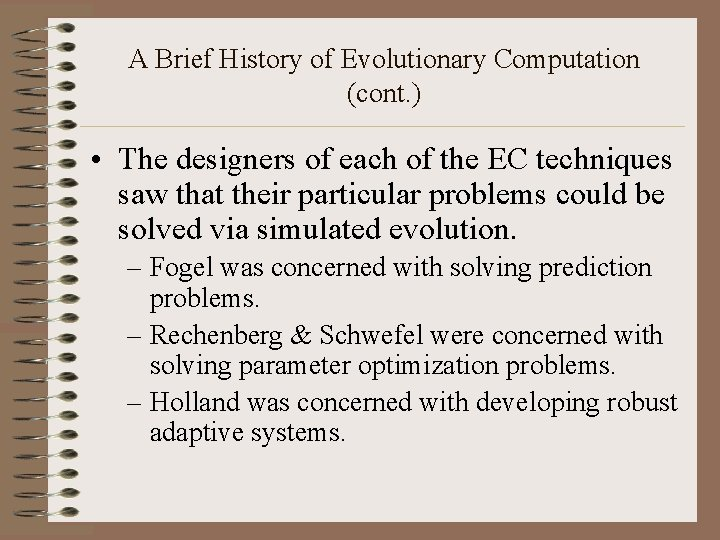A Brief History of Evolutionary Computation (cont. ) • The designers of each of the EC techniques saw that their particular problems could be solved via simulated evolution. – Fogel was concerned with solving prediction problems. – Rechenberg & Schwefel were concerned with solving parameter optimization problems. – Holland was concerned with developing robust adaptive systems.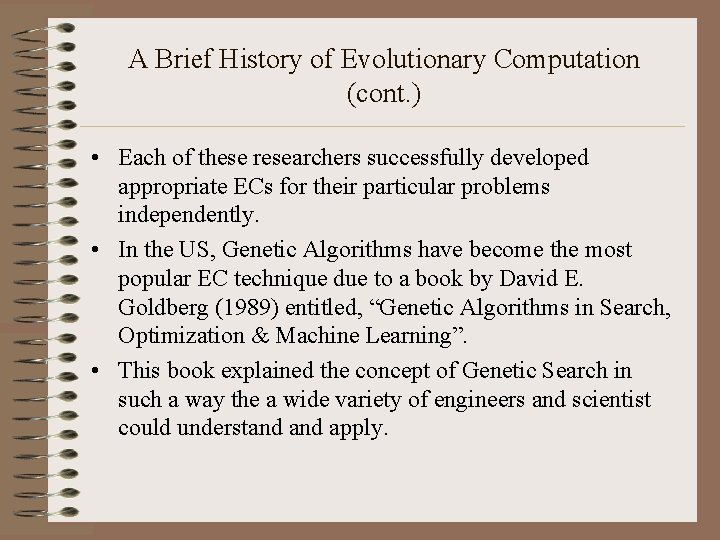A Brief History of Evolutionary Computation (cont. ) • Each of these researchers successfully developed appropriate ECs for their particular problems independently. • In the US, Genetic Algorithms have become the most popular EC technique due to a book by David E. Goldberg (1989) entitled, “Genetic Algorithms in Search, Optimization & Machine Learning”. • This book explained the concept of Genetic Search in such a way the a wide variety of engineers and scientist could understand apply.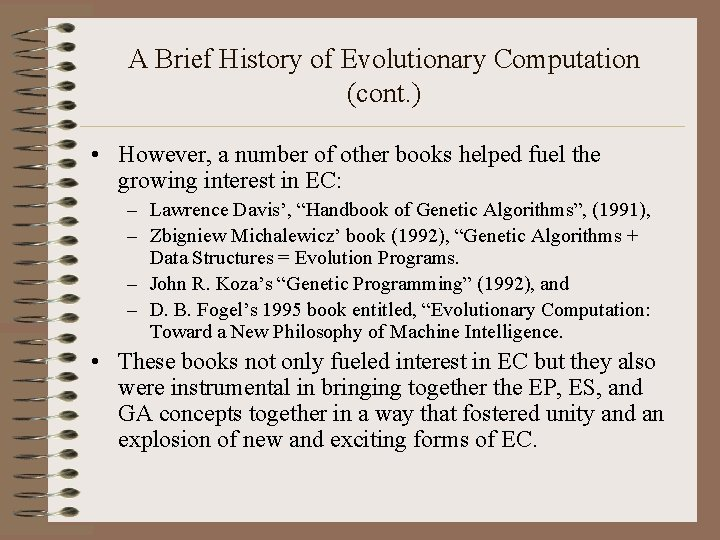A Brief History of Evolutionary Computation (cont. ) • However, a number of other books helped fuel the growing interest in EC: – Lawrence Davis’, “Handbook of Genetic Algorithms”, (1991), – Zbigniew Michalewicz’ book (1992), “Genetic Algorithms + Data Structures = Evolution Programs. – John R. Koza’s “Genetic Programming” (1992), and – D. B. Fogel’s 1995 book entitled, “Evolutionary Computation: Toward a New Philosophy of Machine Intelligence. • These books not only fueled interest in EC but they also were instrumental in bringing together the EP, ES, and GA concepts together in a way that fostered unity and an explosion of new and exciting forms of EC.A Brief History of Evolutionary Computation: The Evolution of Evolutionary Computation • First Generation EC – EP (Fogel) – GA (Holland) – ES (Rechenberg, Schwefel) • Second Generation EC – – Genetic Evolution of Data Structures (Michalewicz) Genetic Evolution of Programs (Koza) Hybrid Genetic Search (Davis) Tabu Search (Glover)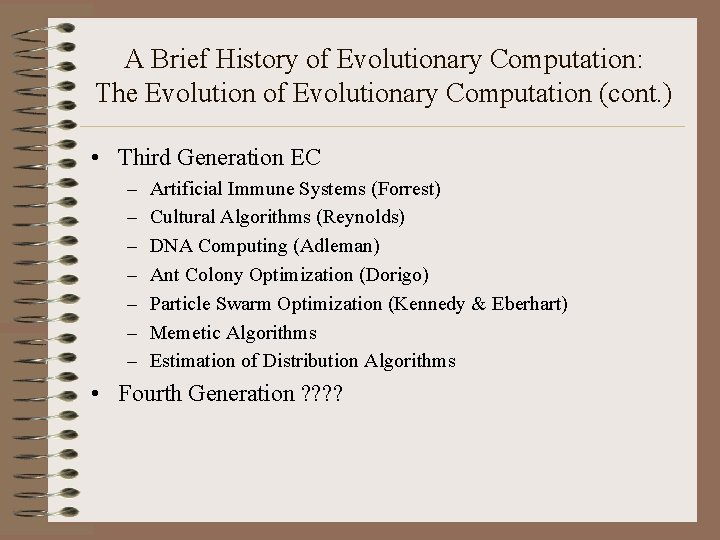A Brief History of Evolutionary Computation: The Evolution of Evolutionary Computation (cont. ) • Third Generation EC – – – – Artificial Immune Systems (Forrest) Cultural Algorithms (Reynolds) DNA Computing (Adleman) Ant Colony Optimization (Dorigo) Particle Swarm Optimization (Kennedy & Eberhart) Memetic Algorithms Estimation of Distribution Algorithms • Fourth Generation ? ?Introduction to Evolutionary Computation: A Simple Example • Let’s walk through a simple example! • Let’s say you were asked to solve the following problem: – – – Maximize: f 6(x, y) = 0. 5 + (sin(sqrt(x 2+y 2))2 – 0. 5)/(1. 0 + 0. 001(x 2+y 2))2 Where x and y are take from [-100. 0, 100. 0] You must find a solution that is greater than 0. 99754, and you can only evaluate a total of 4000 candidate solutions (CSs) • This seems like a difficult problem. It would be nice if we could see what it looks like! This may help us determine a good algorithm for solving it.Introduction to Evolutionary Computation: A Simple Example • A 3 D view of f 6(x, y):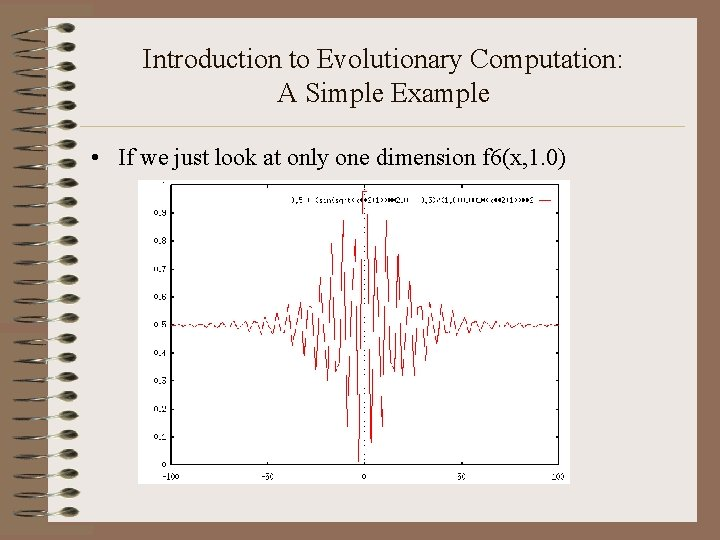Introduction to Evolutionary Computation: A Simple Example • If we just look at only one dimension f 6(x, 1. 0)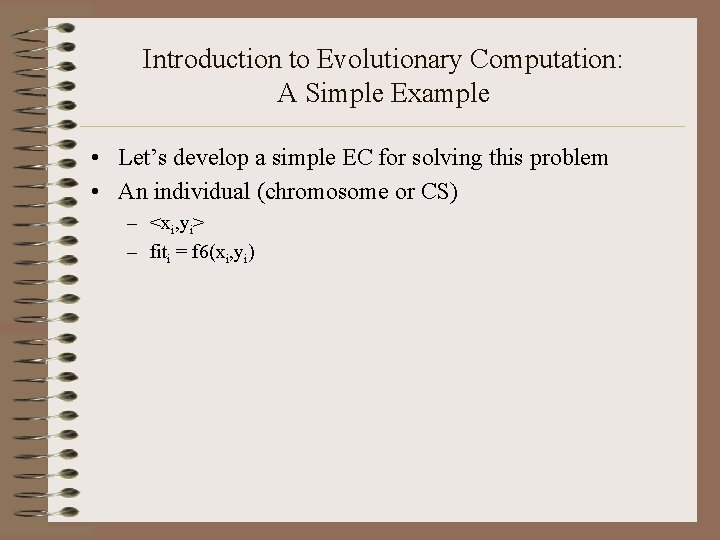Introduction to Evolutionary Computation: A Simple Example • Let’s develop a simple EC for solving this problem • An individual (chromosome or CS) – <xi, yi> – fiti = f 6(xi, yi)Introduction to Evolutionary Computation: A Simple Example Procedure simple. EC{ t = 0; Initialize Pop(t); /* of P individuals */ Evaluate Pop(t); while (t <= 4000 -P){ Select_Parent(<xmom, ymom>); /* Randomly */ Select_Parent(<xdad, ydad>); /* Randomly */ Create_Offspring(<xkid, ykid>): xkid = rnd(xmom, xdad) + Nx(0, ); ykid = rnd(ymom, ydad) + Ny(0, ); fitkid = Evaluate(<xkid, ykid>); Pop(t+1) = Replace(worst, kid); {Pop(t)-{worst}} {kid} t = t + 1; } }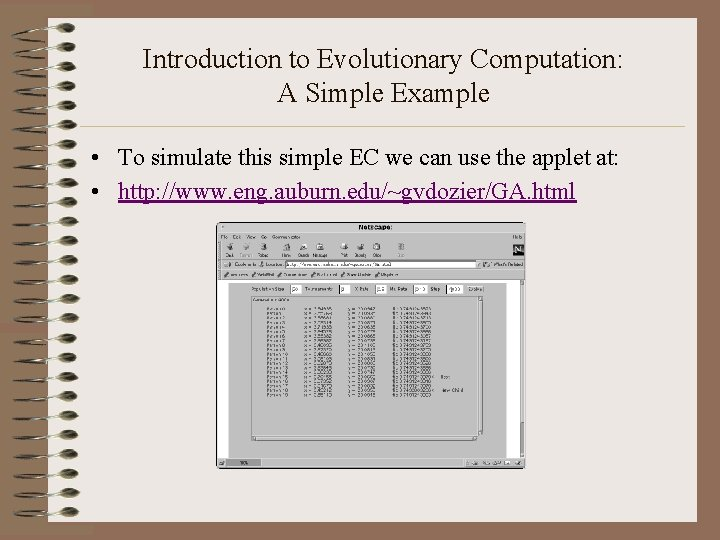Introduction to Evolutionary Computation: A Simple Example • To simulate this simple EC we can use the applet at: • http: //www. eng. auburn. edu/~gvdozier/GA. html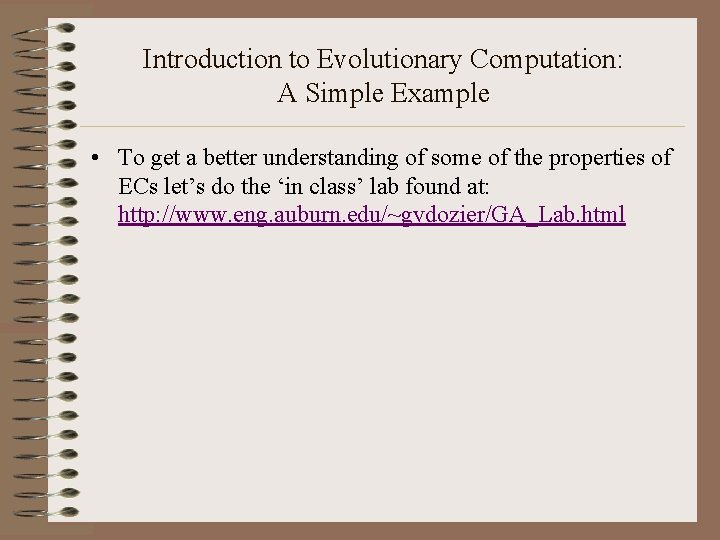Introduction to Evolutionary Computation: A Simple Example • To get a better understanding of some of the properties of ECs let’s do the ‘in class’ lab found at: http: //www. eng. auburn. edu/~gvdozier/GA_Lab. htmlIntroduction to Evolutionary Computation: Reading List 1. 2. 3. Bäck, T. , Hammel, U. , and Schwefel, H. -P. (1997). “Evolutionary Computation: Comments on the History and Current State, ” IEEE Transactions on Evolutionary Computation, VOL. 1, NO. 1, April 1997. Spears, W. M. , De Jong, K. A. , Bäck, T. , Fogel, D. B. , and de Garis, H. (1993). “An Overview of Evolutionary Computation, ” The Proceedings of the European Conference on Machine Learning, v 667, pp. 442 -459. (http: //www. cs. uwyo. edu/~wspears/papers/ecml 93. pdf) De Jong, Kenneth A. , and William M. Spears (1993). “On the State of Evolutionary Computation”, The Proceedings of the Int'l Conference on Genetic Algorithms, pp. 618 -623. (http: //www. cs. uwyo. edu/~wspears/papers/icga 93. pdf)